## R语言 模糊C均值(FCM)聚类分析

FCM(Fuzzy C-Means)算法是一个模糊聚类算法，是对早期硬K-means聚类的一种改性，克服了硬聚类的非此即彼的分类缺点。

FCM属于软聚类，它允许一个数据点可以属于多个类，FCM的价值函数跟K-means非常相似，但其引入了隶属度的概念，使得每个数据点用值在[0,1]的隶属度来确定其属于各个组的程度，其结果是每个数据点对聚类中心的隶属程度（membership），取值在0-1之间，并进行归一化使得总和等于1；因此FCM的思想是使得被划分到同一组下的数据点之间相似性最大，而不同组之间的相似度最小

FCM的C跟K-means的K是相同的概念，是指聚类的数目，Fuzzy是指一个事件的发生程度（模糊相似关系），因此FCM算法需要两个参数：聚类数目C和模糊参数m。一般来说，C要远远小于聚类样本（蛋白/基因）数目，并保证至少大于1；而m（fuzzifier值）是控制算法柔性的参数，用于定义整个数据集的模糊度，一般默认是2，接近于1的话会导致结果接近于K-means聚类，因此不同的C和m会导致聚类结果的不同

FCM聚类算法主要涉及隶属度(隶属矩阵u)、聚类中心v(距离)以及模糊参数m等参数，即在满足隶属度约束条件下，通过迭代计算价值函数的最小值；求约束条件的求极值问题，使用拉格朗日乘子法来构建拉格朗日函数，具体公式推导可看FCM(Fuzzy C-Means)模糊C聚类，算法大致步骤如下：

• 初始化隶属矩阵（满足价值函数的约束条件），计算聚类中心；或者初始化聚类中心
• 迭代计算价值函数，当其小于某个极小值或者前后两次的差值小于某个极小值，则停止
• 更新隶属矩阵，确定聚类结果

``````FCM <- function(x, k, m, iter.max = 1000, con.val = 1e-09){
x <- as.matrix(x)
set.seed(12345)
v0 <- x[sample(nrow(x), 3),]

n <- nrow(x)
d <- matrix(0, n, k)
for (j in 1:k) {
d[, j] = sqrt(rowSums(sweep(x, 2, v0[j, ], "-")^2))
}

u <- matrix(NA, nrow = n, ncol = k)
for (j in 1:k){
for (i in 1:n){
if (any(d[i,] == 0)){
u[i, ] <- rep(1/k, k)
}else{
u[i, j] <- 1/(sum((d[i, j]/d[i, ])^(2/(m - 1))))
}
}
}
v <- t(u^m) %*% x/colSums(u^m)
j <- sum(d^2 * (u^m))

iter <- 0
j_best <- Inf
while((iter < iter.max) && (abs(j - j_best) > con.val)){
j_best <- j
for (j in 1:k) {
d[, j] = sqrt(rowSums(sweep(x, 2, v[j, ], "-")^2))
}

for (j in 1:k){
for (i in 1:n){
if (any(d[i,] == 0)){
u[i, ] <- rep(1/k, k)
}else{
u[i, j] <- 1/(sum((d[i, j]/d[i, ])^(2/(m - 1))))
}
}
}
v <- t(u^m) %*% x/colSums(u^m)
j <- sum(d^2 * (u^m))
iter = iter + 1
}

result <- list()
result\$u <- u
result\$v <- v

return(result)
}
``````

ppclust包的使用说明可以参考Partitioning Cluster Analysis Using Fuzzy C-Means

``````data(iris)
x=iris[,-5]
``````

``````v0 <- inaparc::kmpp(x, k=3)\$v
res.fcm <- fcm(x, centers=v0)

u0 <- inaparc::imembrand(nrow(x), k=3)\$u
res.fcm <- fcm(x, centers=3, memberships=u0)
``````

``````res.fcm <- fcm(x, centers=3, alginitv="hartiganwong", alginitu="imembrand")
``````

``````res.fcm <- fcm(x, centers=3, dmetric="correlation")
``````

``````res.fcm <- fcm(x, centers=3, nstart=5, fixmemb=TRUE)
``````

``````res.fcm <- fcm(x, centers=3, alginitv="hartiganwong", alginitu="imembrand", nstart = 5, fixmemb=TRUE)
res.fcm2 <- ppclust2(res.fcm, "kmeans")
factoextra::fviz_cluster(res.fcm2, data = x,
ellipse.type = "convex",
palette = "jco",
show.legend.text = FALSE)
``````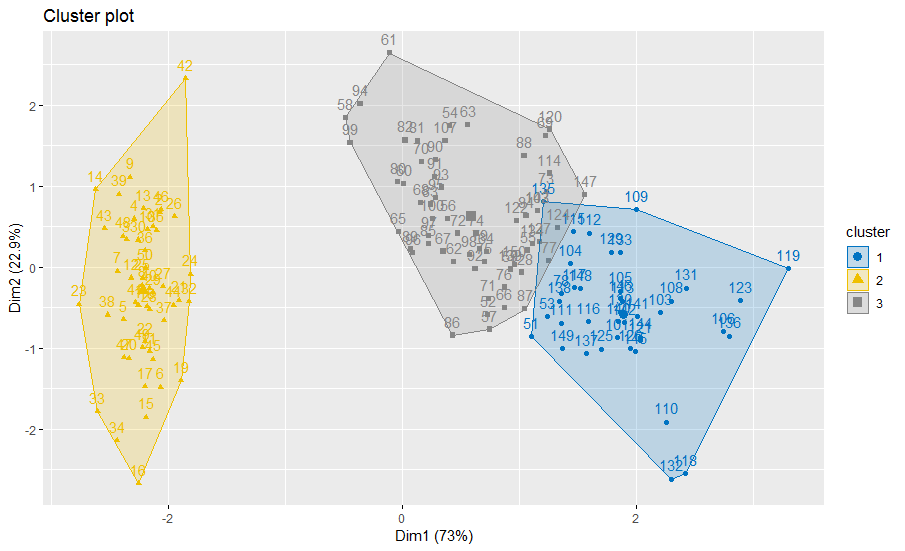``````plotcluster(res.fcm, cp=1, trans=TRUE)
``````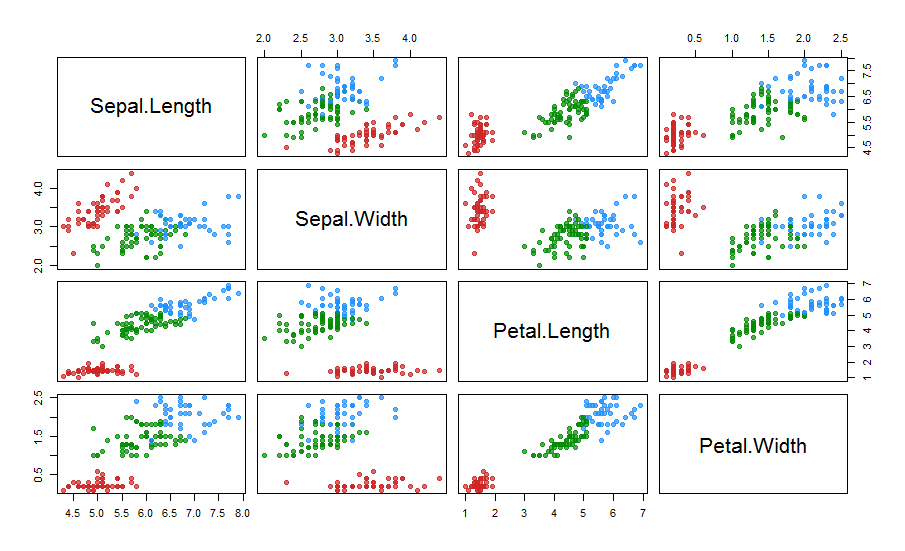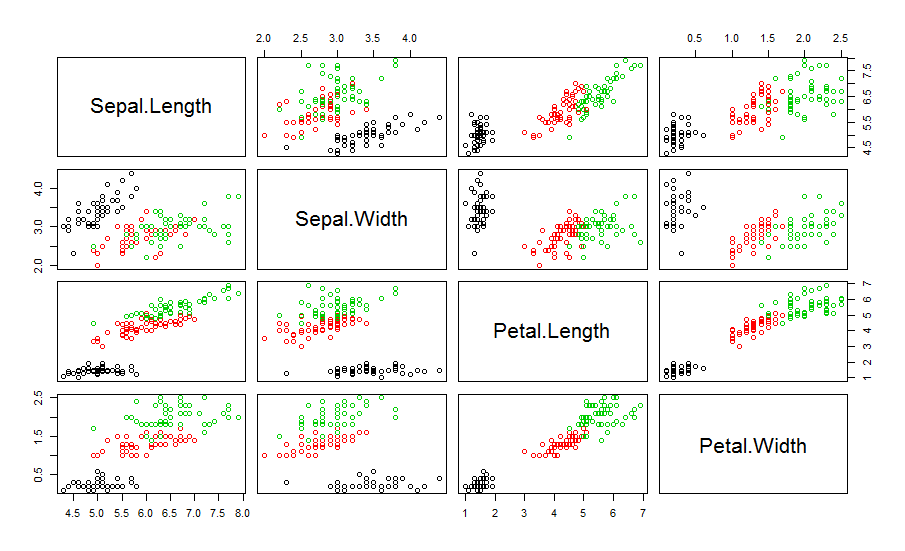``````res.fcm2 <- ppclust2(res.fcm, "fclust")
idxpe <- PE(res.fcm2\$U)
idxpc <- PC(res.fcm2\$U)
``````

``````library(Mfuzz)
data(yeast)

eset <- new("ExpressionSet", exprs = rawdata)
eset <- standardise(eset)
m <- mestimate(eset)
cl <- mfuzz(eset, c = 5, m = m)
mfuzz.plot(eset, cl, mfrow=c(3,2), new.window= FALSE, time.labels = c("CK", "12h", "24h", "48h"))
``````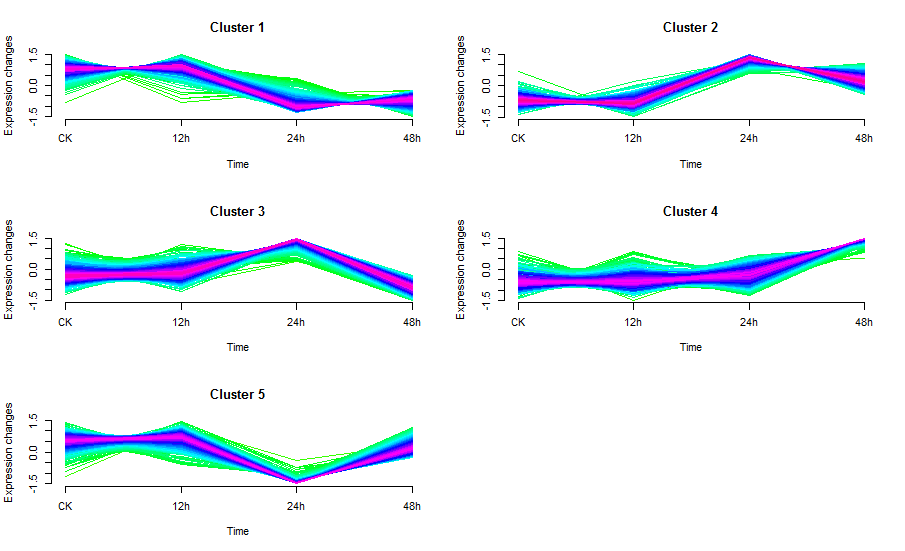``````mat <- matrix(1:2, ncol=2, nrow=1, byrow=TRUE)
l <- layout(mat, width=c(6,1), heights = c(1,5))
mfuzz.plot2(eset, cl, mfrow = NA,
# colo="fancy",
col.sub = "blue",
col = "blue",
cex.main = 2,
single = 2, x11 = FALSE,
time.labels = c("CK", "12h", "24h", "48h"))
mfuzzColorBar(main="Membership", cex.main=1)
``````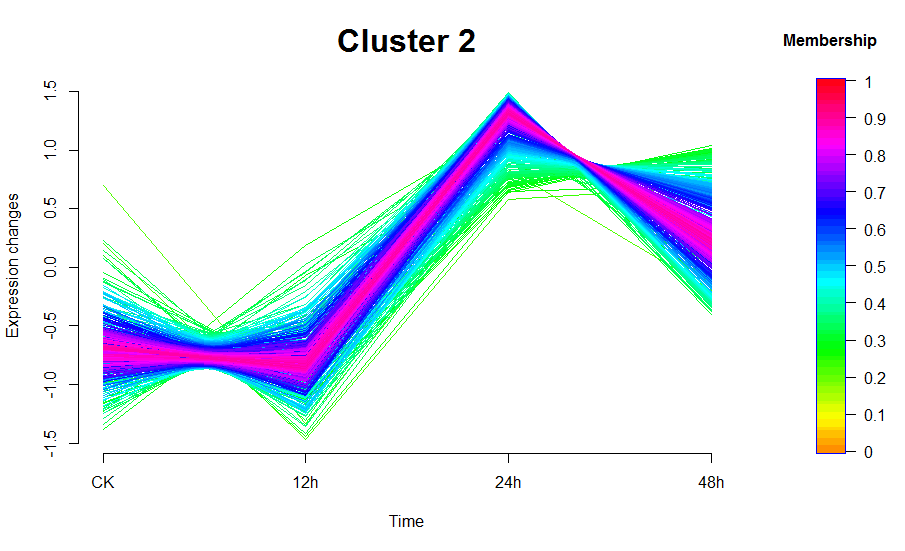``````cl <- cmeans(x = rawdata, centers = 5, iter.max = 1000, method = "cmeans")
resultindexes <- fclustIndex(cl, rawdata, index="all")
resultindexes["xb"]
``````